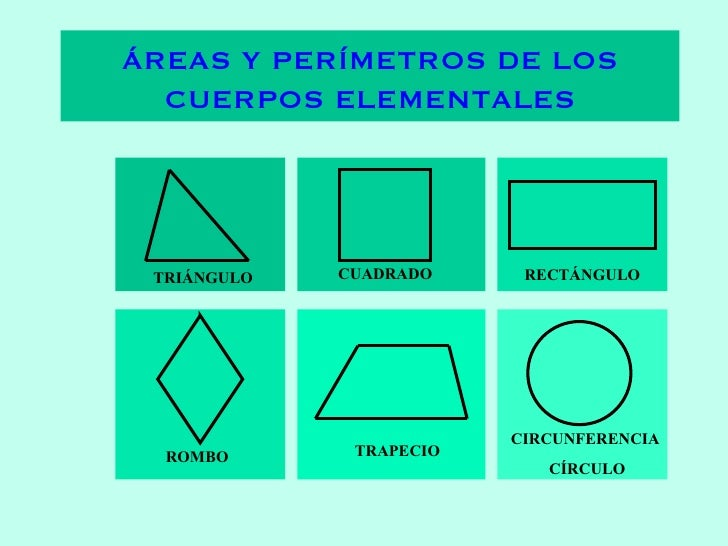Successfully reported this slideshow.Upcoming SlideShare
×

# Perimetrosareas

584 views

Published on

Äreas y perímrtros de los polígonod elementales

Published in: Education
• Full Name
Comment goes here.

Are you sure you want to Yes No• Be the first to comment

• Be the first to like this

### Perimetrosareas

1. 1. ÁREAS Y PERÍMETROS DE LOS CUERPOS ELEMENTALES TRIÁNGULO CUADRADO RECTÁNGULO ROMBO TRAPECIO CIRCUNFERENCIA CÍRCULO
2. 2. TRIÁNGULO área perímetro Pulsa aquí para ver el desarrollo de la fórmula del área Pulsa aquí para ver el desarrollo de la fórmula del perímetro Base por altura partido por dos Suma de los tres lados
3. 3. E J E MP L OS altura h h base b b Área = 3 cm 4 cm 3 cm 2 cm
4. 4. E J E M P L O b a c Perímetro = a + b + c 5 cm 3 cm 4 cm 3 + 5 + 4 = 12 cm
6. 6. E J E MP L O Área = l l Debe ser muy parecida a la del rectángulo Área = a·b a b 5 cm 5 cm
7. 7. E J E M P L O Perímetro = l + l + l + l = 4· l l l 3 cm 3 cm 4·3 = 12 cm
8. 8. RECTÁNGULO área perímetro Pulsa aquí para ver el desarrollo de la fórmula del área Pulsa aquí para ver el desarrollo de la fórmula del perímetro Lado mayor por lado menor Suma de los lados
9. 9. E J E MP L O Área = a · b b a Si los lados fuesen iguales valdría para el cuadrado Área = a·b a b 3 cm 5 cm
10. 10. E J E M P L O Perímetro = a + b + a + b = 2· a + 2· b = 2·( a+b ) b a 3 cm 5 cm 2·(5+3) = 16 cm
11. 11. ROMBO área perímetro Pulsa aquí para ver el desarrollo de la fórmula del área Pulsa aquí para ver el desarrollo de la fórmula del perímetro Diagonal mayor por diagonal menor partido por dos Suma de los lados
12. 12. E J E M P L O Área = D d 8 cm 5 cm
13. 13. E J E M P L O Perímetro = l + l + l + l = 4· l 4·3 = 12 cm l l 3 cm 3 cm
14. 14. TRAPECIO área perímetro Pulsa aquí para ver el desarrollo de la fórmula del área Pulsa aquí para ver el desarrollo de la fórmula del perímetro Semisuma de las bases por la altura Suma de los lados
15. 15. E J E MP L O Si las bases fuesen iguales tendríamos un rectángulo Área = a·b a b h altura b 1 b 2 bases 5 cm 3 cm 2 cm Área =
16. 16. E J E M P L O Perímetro = b 1 + c + b 2 + a 7+3+5+4 = 19 cm a b 2 b 1 c 4 cm 5 cm 7 cm 3 cm
17. 17. círculo circunferencia Pulsa aquí para ver el desarrollo de la fórmula del área Pulsa aquí para ver el desarrollo de la fórmula del perímetro CIRCUNFERENCIA Y CÍRCULO  (pi) por el radio al cuadrado Un balón de playa Será un circulo o será una circunferencia Ni una cosa ni otra Y entonces ¿qué es? Como es posible que no sepa lo que es una esfera Diámetro por   3,14159...
18. 18. E J E M P L O Área = r 10 cm Siempre es un valor aproximado
19. 19. E J E M P L O longitud = r 5 cm Siempre es un valor aproximado